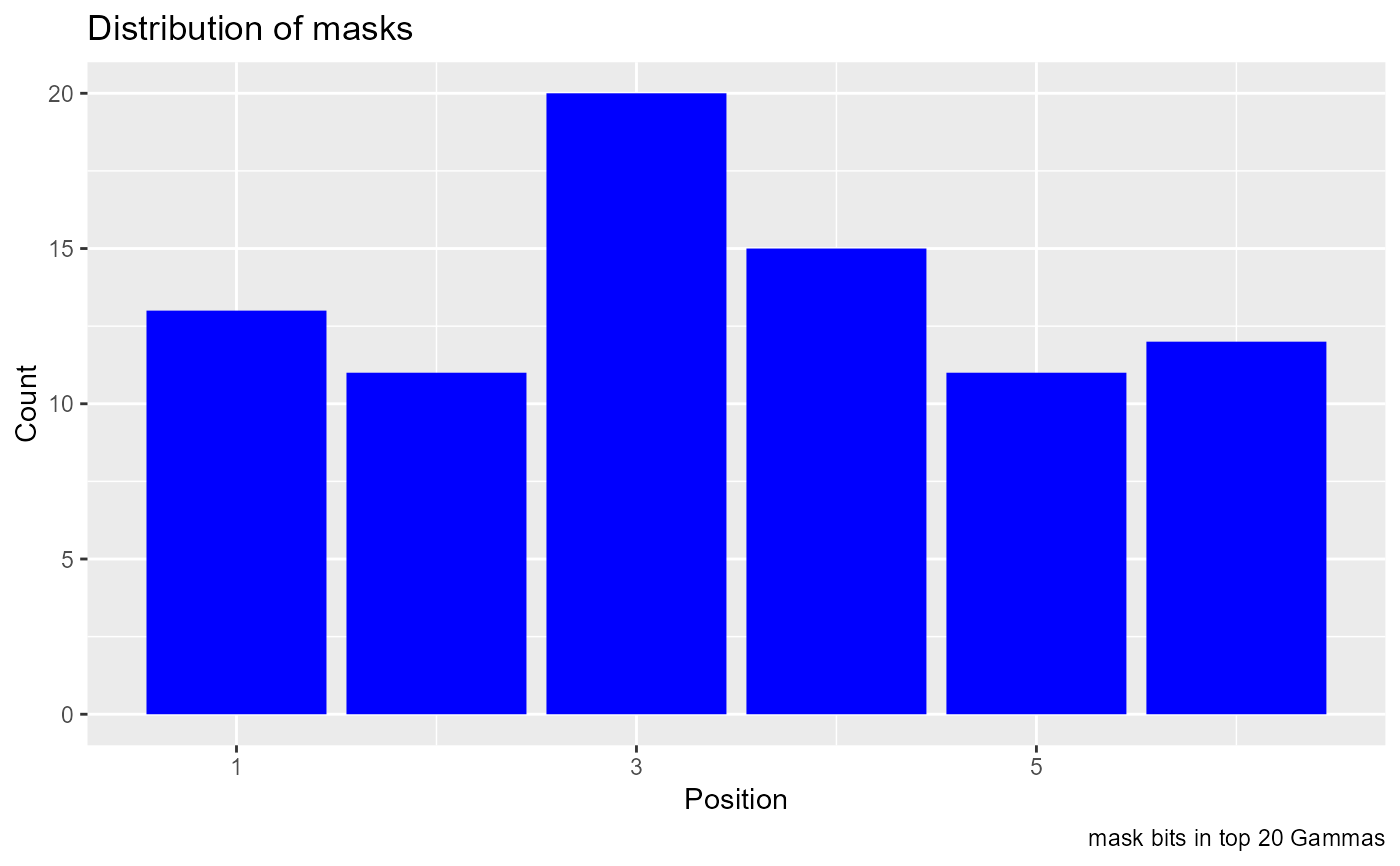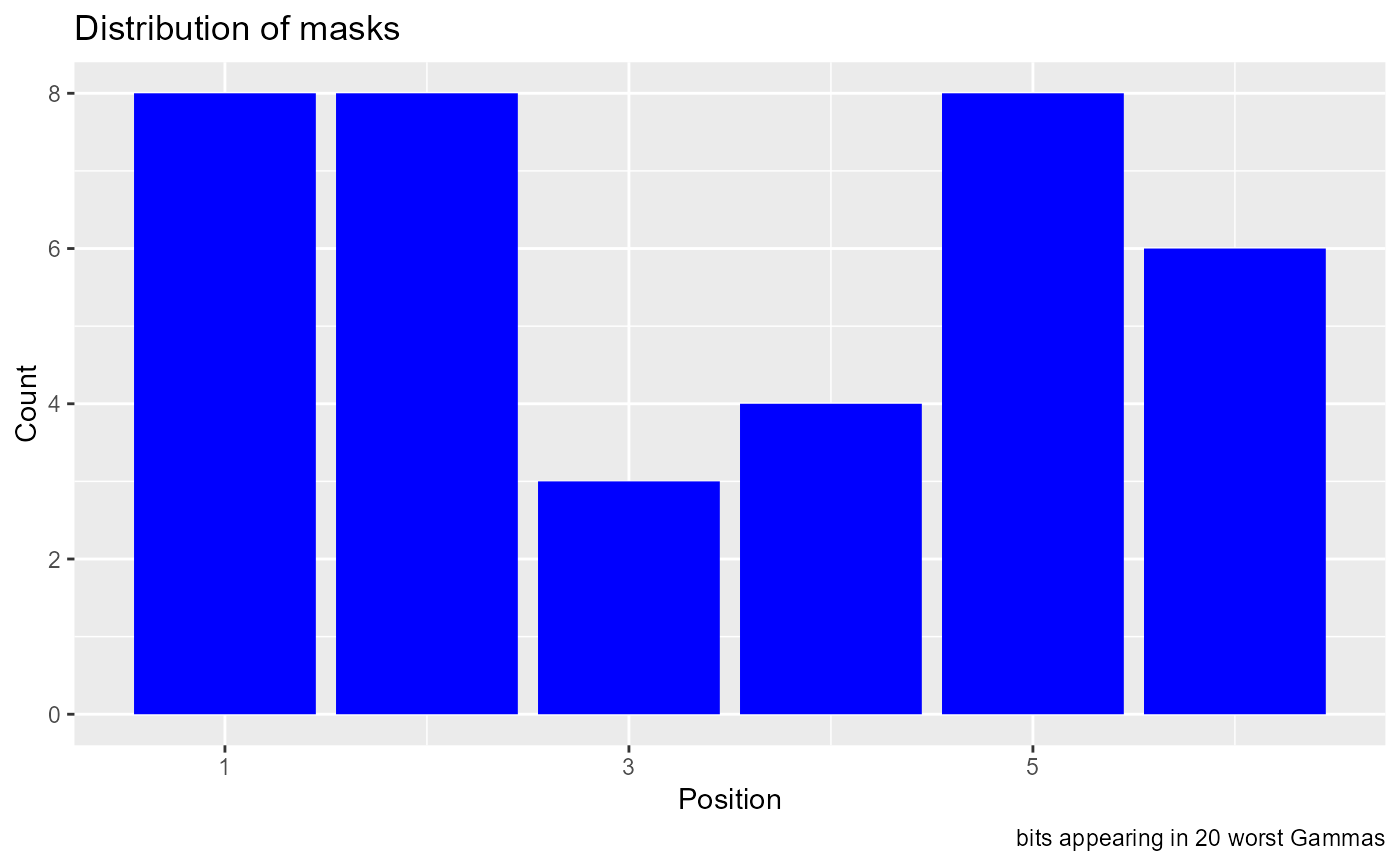Display a histogram of mask bits.

## Usage

mask_histogram(fe_result, dimension, tick_step = 2, caption = "")

## Arguments

fe_result

Output data frame from fe_search. Normally you would filter this by, for example, selecting the top 100 results from that output. If the whole fe_search result was passed in, all of the mask bits would have the same frequency and the histogram would be flat.

dimension

Integer number of effective columns in a mask, ncol of the predictors given to the search

tick_step

Integer, where to put ticks on the x axis

caption

A character string you can use to identify this graph

## Value

A ggplot object, a histogram showing the mask bits used in the fe_search results that are passed to it

## Details

After a full embedding search, it is sometimes useful to see which bits appear in a subset of the masks, for example, the masks with the lowest Gamma values. Filtering of the search results should be done before calling this function, which uses whatever it is given. The histogram can show which predictors are generally useful. For selecting an effective mask it isn't as useful as you might think - it doesn't show interactions between predictors, for mask selection it would only work for linear combinations of inputs.

## Examples

e6 <- embed(mgls, 7)
t <- e6[ ,1]
p <- e6[ ,2:7]
full_search <- fe_search(predictors = p, target = t)